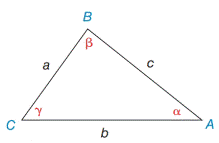Chapter 11.4, Problem 3EElementary Geometry For College St...

7th Edition
Alexander + 2 others
ISBN: 9781337614085

Solutions

Chapter
SectionElementary Geometry For College St...

7th Edition
Alexander + 2 others
ISBN: 9781337614085
Textbook Problem

In Exercises 3 and 4, state the form of the Law of Sines used to solve the problem. Give the answer in a form such as sin   72 ∘ 6.3 = sin   55 ∘ a a) Find β if it is known that a = 5 ,   b = 8 ,  and  α = 40 ∘ . b) Find c if it is known that a = 5.3   α = 41 ∘ ,  and  γ =87 ∘Exercises 1-8

To determine

a.

To Find:

The angle β using the Law of Sines.

Explanation

Formula:

The Law of Sines,

sinαa=sinβb=sinγc

Where α, β, and γ is the acute angle of the triangle, and a, b, and c is the sides of the triangle.

Calculation:

Given,

In ABC, a=5

b=8 and

α=40

To determine

b.

To Find:

The side c using the Law of Sines.

Still sussing out bartleby?

Check out a sample textbook solution.

See a sample solution

The Solution to Your Study Problems

Bartleby provides explanations to thousands of textbook problems written by our experts, many with advanced degrees!

Get Started

Polynomial Inequalities Solve the inequality. 94. x3 3x2 4x + 12 0

Precalculus: Mathematics for Calculus (Standalone Book)

Define sampling with replacement and explain why is it used?

Statistics for The Behavioral Sciences (MindTap Course List)

Find f(n)(x) if f(x) = 1/(2 x).

Single Variable Calculus: Early Transcendentals, Volume I

Which is the best graph of y = 1.5x? (The graph of y = 2x is shown as a dashed curve for comparison.)

Study Guide for Stewart's Single Variable Calculus: Early Transcendentals, 8th